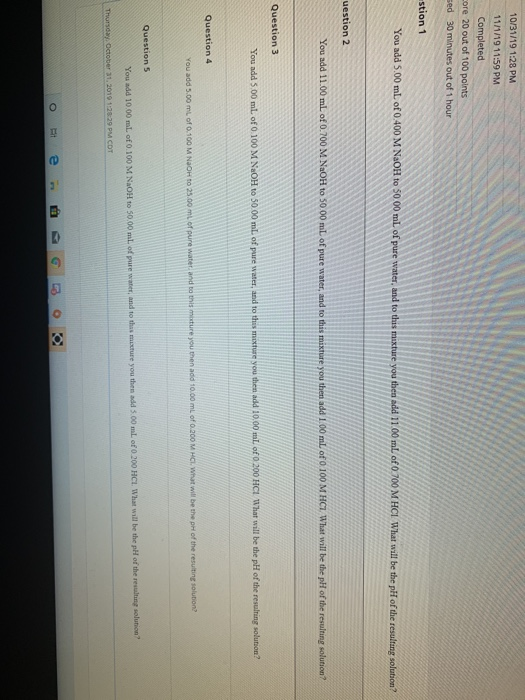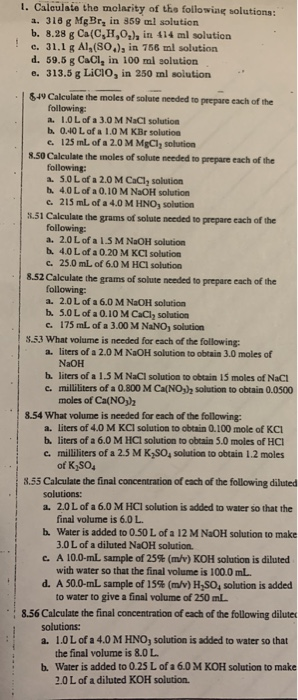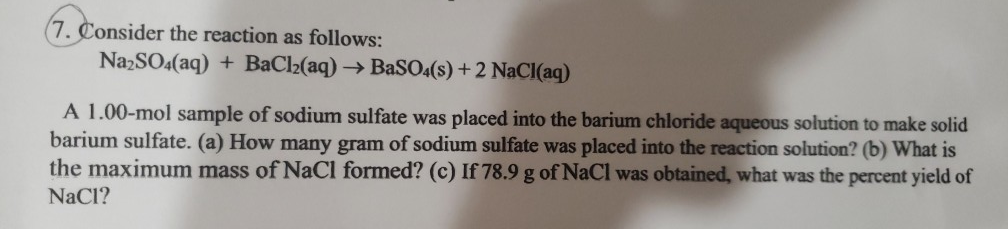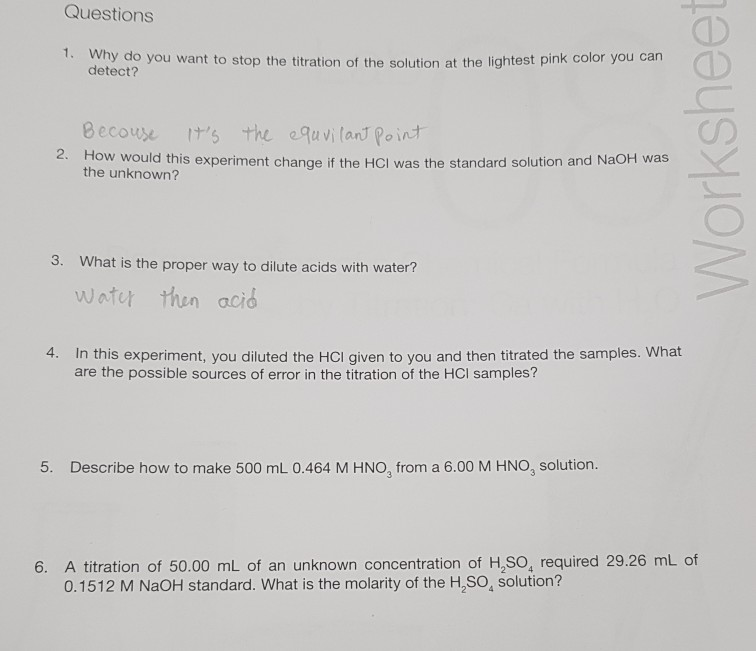# What's V2 in general/how do you calculate it step by step?

This is the question: If I add 25 mL of water to 125 mL of a 0.15 M NaOH solution, what will the molarity of the diluted solution be?

Well volumes are generally additive, and of course the concentration will be diluted.

#### Explanation:

By one of the definitions, $\text{concentration"="Moles of solute"/"Volume of solution} .$

And thus $\text{moles of solute"="concentration"xx"volume of solution}$

And so.....the new concentration will be given by the quotient....

$\frac{125 \times {10}^{-} 3 \cdot \cancel{L} \times 0.15 \cdot m o l \cdot \cancel{{L}^{-} 1}}{125 \times {10}^{-} 3 \cdot L + 25 \times {10}^{-} 3 \cdot L}$

$= 0.125 \cdot m o l \cdot {L}^{-} 1$, i.e. concentration has reduced slightly.

This goes back to the old equality, ${C}_{1} {V}_{1} = {C}_{2} {V}_{2}$, when a given concentration is diluted, the new concentration is readily accessible.

Here we solved for ${C}_{2} = \frac{{C}_{1} {V}_{1}}{V} _ 2$.........

##### Add Answer of: What's V2 in general/how do you calculate it step by step?
Similar Homework Help Questions
• ### Dilution

If 7.0 mL of 6.0 M NaOH are diluted with water to a volume of 400 mL, what is the molarity of the resulting solution?

• ### General chemistry

The molar concentration of hydrochloric acid (HCl) in a solution commercially used to remove boiler scale from plumbing can be determined by titration with NaOHsolution. The reaction of NaOH with HCl is shown in the following equation:HCl(aq) + NaOH(aq) ---> H2O(l) + NaCl(aq)consider the following example. After we transfer a 10.00-mL sample of HCl solution into a 125-mL Erlenmeyer flask, we add 50 mL of distilled water and 3 drops ofphenolphthalein indicator solution. The resulting solution requires 44.00 mL of...

• ### Molarity

If 166 mL of 1.00 M NaOH is diluted to 1.00 L, what is the molarity of the resulting solution?

• ### Titration

25 mL of an unknown vinegar solution is diluted with 225 mL of water. 50 mL of this mixed solution is put into another beaker and diluted with 50 mL of water. In orderto figure out the molarity, what is the volume you would use?

• ### ok so i keep getting these wrong idk why but im doing them write you find the poh then subtract from 14 right? or is it...ok so i keep getting these wrong idk why but im doing them write you find the poh then subtract from 14 right? or is it just the poh 10/31/19 1:28 PM 1171/19 11:59 PM Completed core 20 out of 100 points sed 30 minutes out of 1 hour stion 1 You add 5.00 ml of 0.400 M NaOH to 5000 mL of pure water, and to this mixture you then add 11.00 ml of 0 700 M HCL What...

• ### 1. Calculate the molarity of the following solutions: a. 318 g Mg Br, in 859 ml...1. Calculate the molarity of the following solutions: a. 318 g Mg Br, in 859 ml solution b. 8.28 g Ca(C,H,O,), in 414 ml solution c. 31,1 g Al,(80.), in 766 ml solution d. 59.8 g CaCl, in 100 ml solution e. 313.5 g LICIO, in 250 ml solution | 849 Calculate the moles of solute needed to prepare each of the following: 2. LOL of a 3.0 M NaCl solution b. 0.40 L of a 1.0 M KBr solution...

• ### I will rate you well!

A 188 mL sample of a 1.4 M sucrose solution is diluted to 550 mL.What is the molarity of the diluted solution?

• ### Properties of Solutions

Hello,My question is:A 15 ml solution of potassium nitrate was diluted to 125 ml and 25 ml of this solution was then diluted to 1 L. The concentration of the final solution is 0.00383.What was the concentration of the initial solution>Thanks.

• ### (7. Consider the reaction as follows: Na2SO4(aq) + BaCl2(aq) → BaSO4(s) + 2 NaCl(aq) A 1.00-mol sample of sodium...(7. Consider the reaction as follows: Na2SO4(aq) + BaCl2(aq) → BaSO4(s) + 2 NaCl(aq) A 1.00-mol sample of sodium sulfate was placed into the barium chloride aqueous solution to make solid barium sulfate. (a) How many gram of sodium sulfate was placed into the reaction solution? (b) What is the maximum mass of NaCl formed? (c) If 78.9 g of NaCl was obtained, what was the percent yield of NaCl? 3. How many liters of 0.186 M of NaOH (aq)...

• ### Questions 1. Why do you want to stop the titration of want to stop the titration...Questions 1. Why do you want to stop the titration of want to stop the titration of the solution at the lightest pink color you can detect? Because it's the equvilant point 2. How would this experiment change if the HCI was the standard solution and NaOH was the unknown? 3. What is the proper way to dilute acids with water? water then acid 4. In this experiment, you diluted the HCI given to you and then titrated the samples....

Need Online Homework Help?